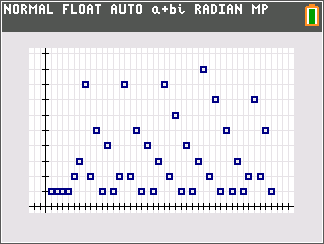## Tuesday, July 28, 2015

### Extracting the First Digit

NOTICE:  The author does not use third-party features on this blog.   In order to comply with European Union laws, Google added a notice to explain Google’s use of certain Blogger and Google cookies.

Extracting the First Digit

Define the function FD(x) as:

FD(x) = int(x/10^(int log |x|))

If x > 0 (positive), this form can also be used:

FD(x) = int(x/10^(int log x))

The function int represents the integer part function (iPart on TI calculators, IP on most HP calculators).    This function extracts the first digit (most significant digit) of x, where the domain is:

FD(x) = 0  if -1 < x < 1,
FD(x) is Undefined when x = 0, and
FD(x) ϵ {1, 2, 3, 4, 5, 6, 7, 8, 9} for all other x.

Examples:

FD(0) = 0
FD(26) = 2
FD(324) = 3
FD(9273) = 9

FD(23) = 2
FD(23.28109) = 2
FD(-23) = 2
FD(-23.28109) = 2

FD(π) = 3
FD(1/2) = 0

Here are some graphs of FD(x), FD(e^x), FD(x^2), FD(x^2-2*x+2), and FD(e^(x-5)+1)  for integers x from 1 to 40.  This was done using a stat plot on a TI-84 Plus CE.FD(x)FD(e^x) – this looks like a dragon.FD(x^2-2*x+2)FD(e^(x-5)+1) - a party? Something to explore… Eddie This blog is property of Edward Shore.  2015.